A couple of weeks ago, Alain Connes and Katia Consani arXived their paper “On the notion of geometry over“. Their subtle definition is phrased entirely in Grothendieck‘s scheme-theoretic language of representable functors and may be somewhat hard to get through if you only had a few years of mathematics.I’ll try to give the essence of their definition of an affine scheme over(and illustrate it with an example) in a couple of posts. All you need to know is what a finite Abelian group is (if you know what a cyclic group is that’ll be enough) and what a commutative algebra is. If you already know what a functor and a natural transformation is, that would be great, but we’ll deal with all that abstract nonsense when we’ll need it.

So take two finite Abelian groups A and B, then a group-morphism is just a map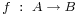preserving the group-data. That is, f sends the unit element of A to that of B and f sends a product of two elements in A to the product of their images in B. For example, if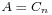is a cyclic group of order n with generator g andis a cyclic group of order m with generator h, then every groupmorphism from A to B is entirely determined by the image of g let’s say that this image is. But, as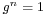and the conditions on a group-morphism we must have that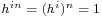and therefore m must divide i.n. This gives you all possible group-morphisms from A to B.

They are plenty of finite abelian groups and many group-morphisms between any pair of them and all this stuff we put into one giant sack and label it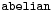. There is another, even bigger sack, which is even simpler to describe. It is labeled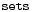and contains all sets as well as all maps between two sets.

Right! Now what might be a mapbetween these two sacks? Well, F should map any abelian group A to a set F(A) and any group-morphismto a map between the corresponding setsand do all of this nicely. That is, F should send compositions of group-morphisms to compositions of the corresponding maps, and so on. If you take a pen and a piece of paper, you’re bound to come up with the exact definition of a functor (that’s what F is called).

You want an example? Well, lets take F to be the map sending an Abelian group A to its set of elements (also called A) and which sends a groupmorphismto the same map from A to B. All F does is ‘forget’ the extra group-conditions on the sets and maps. For this reason F is called the forgetful functor. We will denote this particular functor by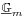, merely to show off.

Luckily, there are lots of other and more interesting examples of such functors. Our first class we will call maxi-functors and they are defined using a finitely generated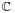-algebra R. That is, R can be written as the quotient of a polynomial algebra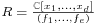by setting all the polynomials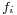to be zero. For example, take R to be the ring of Laurant polynomials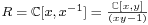Other, and easier, examples of-algebras is the group-algebraof a finite Abelian group A. This group-algebra is a finite dimensional vectorspace with basis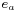, one for each elementwith multiplication rule induced by the relationswhere on the left-hand side the multiplication . is in the group-algebra whereas on the right hand side the multiplication in the index is that of the group A. By choosing a different basis one can show that the group-algebra is really just the direct sum of copies ofwith component-wise addition and multiplicationwith as many copies as there are elements in the group A. For example, for the cyclic groupwe haveThe maxi-functor asociated to a-algebra R is the functorwhich assigns to a finite Abelian group A the set of all algebra-morphismfrom R to the group-algebra of A. But wait, you say (i hope), we also needed a functor to do something on groupmorphisms. Exactly, so to f we have an algebra-morphism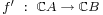so the functor on morphisms is defined via composition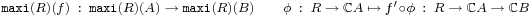So, what is the maxi-functor? Well, any-algebra morphismis fully determined by the image ofwhich must be a unit in. That is, all components of the image ofmust be non-zero complex numbers, that is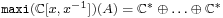where there are as many components as there are elements in A. Thus, the sets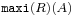are typically huge which is the reason for the maxi-terminology.

Next, let us turn to mini-functors. They are defined similarly but this time using finitely generated-algebras such as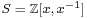and the integral group-ringsfor finite Abelian groups A. The structure of these inegral group-rings is a lot more delicate than in the complex case. Let’s consider them for the smallest cyclic groups (the isos below are only approximately correct!)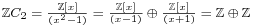For a-algebra S we can define its mini-functor to be the functorwhich assigns to an Abelian group A the set of all-algebra morphisms. For example, for the algebrawe have thatthe set of all invertible elements in the integral group-algebra. To study these sets one has to study the units of cyclotomic integers. From the above decompositions it is easy to verify that for the first few cyclic groups, the corresponding sets areand. However, in general this set doesn’t have to be finite. It is a well-known result that the group of units of an integral group-ring of a finite Abelian group is of the formwherewhereis the number of elements of A,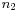is the number of elements of order 2 and c is the number of cyclic subgroups of A. So, these sets can still be infinite but at least they are a lot more manageable, explaining the mini-terminology.

Now, we would love to go one step deeper and define nano-functors by the same procedure, this time using finitely generated algebras over, the field with one element. But as we do not really know what we might mean by this, we simply define a nano-functor to be a subfunctor of a mini-functor, that is, a nano-functor N has an associated mini-functorsuch that for all finite Abelian groups A we have that.

For example, the forgetful functor at the beginning, which we pompously denotedis a nano-functor as it is a subfunctor of the mini-functor.

Now we are allmost done : an affine-scheme in the sense of Connes and Consani is a pair consisting of a nano-functor N and a maxi-functorsuch that two rather strong conditions are satisfied :

• there is an evaluation ‘map’ of functors• this pair determines uniquely a ‘minimal’ mini-functorof which N is a subfunctor

of course we still have to turn this into proper definitions but that will have to await another post. For now, suffice it to say that the pairis a-scheme with corresponding uniquely determined mini-functor, called the multiplicative group scheme.

Continued herePrint This Post

1.Do all your undergrads know about Maschke’s theorem? How lucky of them, I only started learning about these things once I finished my degree and started taking PhD courses. Do you have a reference for that result about the units on, I’d guess Passman’s is the go-to place, but just in case…

2.As to Maschke : yes, in Antwerp they learn this in their second bachelor year. For the result on units in integral group rings, perhaps the best place are Sehgal’s papers on the subject, for example it’s mentioned in this paper.

3.How do the evaluation morphisms in SoulÃ© gadgets produce the natural transformation in C-C framework? I see how we can define for every object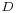and any pointwe can produce a set map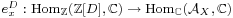via. According to C-C (Lemma 2.4) that induces a complex algebra map, but I don’t quite see how. Only thing I can think about is assuming that for every, we could defineas an element insuch that for every characterof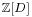we have, where, but that leaves the problem on proving existence and uniqueness of such an element of.

Or am I getting something wrong and it follows from some adjunction properties? I find all of it a bit confusing, since we are mixing up complex algebra maps, ring homomorphisms and set maps… any ideas?

4.On the technical side, WP keeps sanitizing the backslashes and trying to convert underscores to “em” tags inside the tex labels.

5.isn’t the C-C-lemma 2.4 claim merely what’s called the ‘duality theorem’? Saying that there is a natural 1-1 correspondence (actually an anti-equivalence so the direction of arrows reverses) between polynomial maps between affine varieties and algebra maps between the coordinate rings. Hom_C(A_X,C) are just the points of the affine variety X, that is, the affine variety X. Similarly, tensoring up the left side with C gives Hom_C(C[D],C), so the points of the (reducible) variety max(C[D]).

6.Maybe I am a hopeless case, but don’t see what does that have to do with the problem… first of all, X is just a functor coming from a gadget, not an affine variety, I guess you mean points of Spec(A_X)? Second, the map we have (e_x^D in my above notation) is in principle just a set map, there is no reason for it to be a bijection (or there is?), even if it was, “tensoring by C” on the left hand side is a bit strange, since from the beginning we have mappings landing in C, not in Z, so in the second part of the Hom we would obtain a tensor product of C with itself over the integers, which is a sort of big thing Am I missing something obvious or just not understanding the result?

7.i had to look up the paper again. a soule gadget has the property that for each Z-algebra morphism Z[D]–>C and every x in X(D) there is a C-algebra morphism A_X–>C.

Now, any Z-algebra morphism Z[D]–>C determines (and is determined by) a group-morphism D–>C^* (that is, by a character) and as such extends to a C-algebra morphism C[D]–>C.

Max(C[D]) is the set of all characters of D. Lets call Max(A_X) the variety of all 1-dml reps of A_X (exists even when A_X is noncommutative). In fact, Max(A_X) = Max(A_X/[A_X,A_X]) so is the affine variety of a commutative algebra (affine, if A_X is supposed to be affine).

The soule-condition determines for each x in X(D) a map from Max(C[D])–>Max(A_X) and this is a polynomial map because it just sends a finite number of points to points of the variety corresponding to the commutative ring B (A_X mod commutators). So, by duality this gives an algebra map of commutative algebras B —> C[D]. The corresponding C-algebra map of lemma 2.4 is then the composition A_X –>> B –> C[D].

So, this then gives the map of sets X(D)–>Hom_C(A_X,C[D]).

8.Ok, thanks, I got it now, I was thinking in the wrong direction… Actually, the same thing is true in a more general setting, namely, if we have a set map
e: Hom_Z(R,C) –> Hom_C(A,C) then there is an algebra map from A to R_C, no need of R being a group ring or anything (maybe just flatness to avoid nasty torsion killing on the tensor product). The way of constructing such a map is as follows: Take a character s: R_C –> C, then by composing with the natural inclusion R –> R_C it induces a character s’: R –> C, upon which we can apply our evaluation map e, getting a map e’: Hom_C(R_C,C) –> Hom_C(A,C) defined by e’(s) := e(s’). This is a map between the corresponding maximal spectra, and thus induces an algebra map between the algebras, in the opposite direction.

I feel so stupidly frustrated now… first I was frustrated by not being able to solve the problem, and now because I feel that I should have realized before! Does math keep being like this forever, or it gets any better when you get a permanent position?